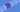# Python - Functions

### 2020-04-21

Our topic in this lesson is a common pattern most programming languages called **functions. **Functions are a way to create a piece of logic that we want to run multiple times.

For example, let's say we have want to compute how much money we will make if we work at a particular job for a number of years. We can create a function to compute this value for us.

``````def calculateMoney(numberOfYears):
return 60000 * numberOfYears # 60000 is the salary for this job

money = calculateMoney(1)
print(money) # prints 60000

moeny = calculateMoney(3)
print(money) # prints 180000``````

We use the def keyword to declare or define a function. Then we follow that with the name of the function. In this case our function is called calculateMoney. The name is followed by open and closed parenthesis with items called **arguments, **and ends with a colon.

In this function we only have one argument called **numberOfYears. **This allows us or anyone using our function to pass in a value for numberOfYears. You can see how we use it above by provding a 1 and 3 value.

The benefit to using a function is that if we need to change the logic, we only need to change it in one place. For example, let's say the salary increased to 80,000. We only need to change this in one place.

``````def calculateMoney(numberOfYears):
return 80000 * numberOfYears # 80000 is the salary for this job

money = calculateMoney(1)
print(money) # prints 80000

money = calculateMoney(3)
print(money) # prints 240000``````

That's it! And the code keeps working with our new salary. We can see here that functions allow us to create chunks of logic to be reused in many places.

Let's end by adding one more argument to our function.

``````def calculateMoney(numberOfYears, salary):
return salary * numberOfYears # 80000 is the salary for this job

jobSalary = 80000
calculateMoney(1, jobSalary) # prints 80000
calculateMoney(3, jobSalary) # prints 240000``````

In this example, we added an additional argument to our function by using a comma. We now allow for passing the value of the salary into our equation. You can check out this example online at https://pyfiddle.io/fiddle/a863b9e4-5a08-49d6-9816-fd5f1fcbc0a6/

GoTea - KoalaTea